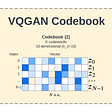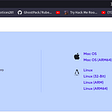Algorithms and Explanations with Code

Supervised Learning is a type of machine learning used to “train models from labelled training data”. It allows use to predict the output for future or unseen data.

Supervised learning cab be used in this case as all the data is “labelled data”.

# Supervised Learning Flow (Understanding Algorithms )

Training data consist of input also known as “Features” and output also known as “Label”.

# Testing, Testing, Prediction

We divide “Data” into two part :

Training Data : We give roughly 70% data from our raw data file to machine learning algorithm on which machine trains itself.

Test Data : We give roughly 30% data from our raw data file to machine learning algorithm on which machine test itself.

The total prediction data is randomly distributed between different samples and a sample is chooses randomly.

## New Data —> Predictive Model ( use the learn algorithm y + f (x) to predict production data ) —> Prediction Outcome

Algorithm prediction can be improved by providing more training data and capacity or by algorithm redesign.

# Types of Supervised Learning

There are two types of Supervised Learning :

Classification

Regression

# Regression

• In Regression algorithm the output has a. “continuous value” that is not restricted to defined separated value.
• Regression predicts a value as close to the actual output value as possible and evaluates it by calculating error value.
• The error value is inversely proportional to accuracy of model.

## When To Use

Classification involves predicting categorical class labels . If the target variable is categorical (class) then use classification algorithm . It is applied when the output has finite and discrete values.

Regression involves finding the best-fit straight line. through the plotted points. If the target variable is a continuous numeric variable then use regression algorithms. We can predict a continuous dependent variable from a number of independent variables ( y = w*x + b ). This shows the relationship between ‘y’ and ‘x’ .

# Types of Supervised Learning Regression Algorithm :

Linear Regression : Linear Regression is a statistical model used to predict the relationship between independent and dependent variable denoted by x and y respectively.

## It examines 2 factors

Q 1) How closely are x and y related ?

Answer – Linear Regression gives a number between -1 and 1 indicating the. strength of the correlation between two variables .

0 : No correlation

1 : Positively correlated

-1 : Negatively correlated

Q 2) Prediction ?

Answer – When the relationship between x and y is known it uses this to predict. further values of y for a value of x. This is done by fitting a regression line and is represented by linear equation. [ y = a*x + b ]

## Code for importing and fitting the model

from sklearn.linear_model import LinearRegression

variable_name = LinearRegression ( )

regressor.fit(independent_data_train,dependent_data_train)

Multiple Linear Regression : Multiple Linear Regression is used to predict the outcome of the response variable through several explanatory variables and to model the relationships between them.

[ y = m1*x1 + m2*x2 + m3*x3 + m4*x4 + m5*x5 + m6*x6 +__________________ + mn*xn +c

## Code for importing and fitting the model (uses LinearRegression model)

from sklearn.linear_model import LinearRegression

variable_name = LinearRegression ( )

regressor.fit(independent_data_train,dependent_data_train)

Polynomial Regression : Polynomial Regression relationship between the dependent variable y and the independent variable x is modeled as an nth degree of polynomial in x.

## Code for importing and fitting the model

from sklearn.linear_model import LinearRegression

variable_name = LinearRegression ( )

variable_name.fit(independent dataset,dependent dataset)

## Calling (polynomial feature) class which is simple a class that will gives use a tool to include polynomial terms to Linear Regression.

from sklearn.preprocessing import PolynomialFeatures

variable_name1 = PolynomialFeatures (degree = number of degree you want)

variable_name2 = variable_name1.fit_transform(independent dataset)

## Note – It will be a transfer tool to transfer MatrixFeature (Independent dataset) to independent set of polynomial model.

variable_name3 = LinearRegression( )

variable_name3.fit(variable_name2, dependent dataset)

## Note – This will fit polynomial model to LinearRegression

Logistic Regression : Logistic Regression is used to estimate discrete values ( binary value like 0/1 , yes/no, true/false) based on a given set of independent variable(s).

## Code for importing and fitting the model

from sklearn.linear_model import LogisticRegression

variable_name = LogisticRegression ( random_state =0 )

variable_name.fit(independent_data_train,dependent_data_train)

Decision Tree : Decision tree make sequential hierarchical decisions about the outcome variable based on predictor data.

Root Node : The entire population or sample that further gets divided .

Splitting : Division of nodes into two or more. sub-nodes.

Decision Node : A sub-node spilts into further sub-nodes.

Leaf/Terminal Node : Node that does not split.

Branch/Sub-Trees : A subsection of the entire tree .

Parent Node : A node which is divided into sub-nodes are the child node.

## Code for importing and fitting the model

from sklearn.tree import DecisionTreeRegressor

variable_name = DecisionTreeRegressor( random_state =0 )

variable_name.fit(independent_data_train,dependent_data_train)

Random Forest : Random Forest is the ensemble of decision tree given better prediction and accuracy than a decision tree.

## Code for importing and fitting the model

from sklearn.ensemble import RandomForestRegressor

variable_name = RandomForestRegressor(n_estimator =300, random_state =0 )

variable_name.fit(independent_data_train,dependent_data_train)

## note – n_estimator = number of tree sets.

Naive Bayes : Naive Bayes is based on Baye’s theorem and works with an assumption that features are independent.

## Code for importing and fitting the model

from sklearn.naive_bayes import GaussianNB

variable_name = GaussianNB

variable_name.fit(independent_data_train,dependent_data_train)

Support Vector Machines : Support Vector Machines draws hyperplane in a feature space that separates instances into different categories with margins in between as far apart as possible.

## Code for importing and fitting the model

from sklearn.svm import SVC

variable_name = SVC (kernel = ‘your choice depends on model’ ,

random_state = 0)

variable_name.fit(independent_data_train,dependent_data_train)

Note – kernel can be ‘linear’ , ‘rbf’ or any other based on your model.

--

--

--

## More from Kartikeya Mishra

All about new technology in fun and easy way so that you can be confident in it and make your own piece of work using this knowledge !

Love podcasts or audiobooks? Learn on the go with our new app.

## Next generation of edge computing: AI, microservices and container orchestration## Training a Computer to Classify Distinct Images Using a Convolutional Neural Network## THE BLOG Part: Understanding words## Quora Insincere Questions Classification## Optimization in ML/DL— 1 (Gradient Descent and its Variant)## Understanding how DALL-E mini works## Stock Price Prediction## Kartikeya Mishra

All about new technology in fun and easy way so that you can be confident in it and make your own piece of work using this knowledge !

## How to find Common and Uncommon Values between 2 Lists in UiPath## Learn to Love Brackish## Unsupervised Machine Learning